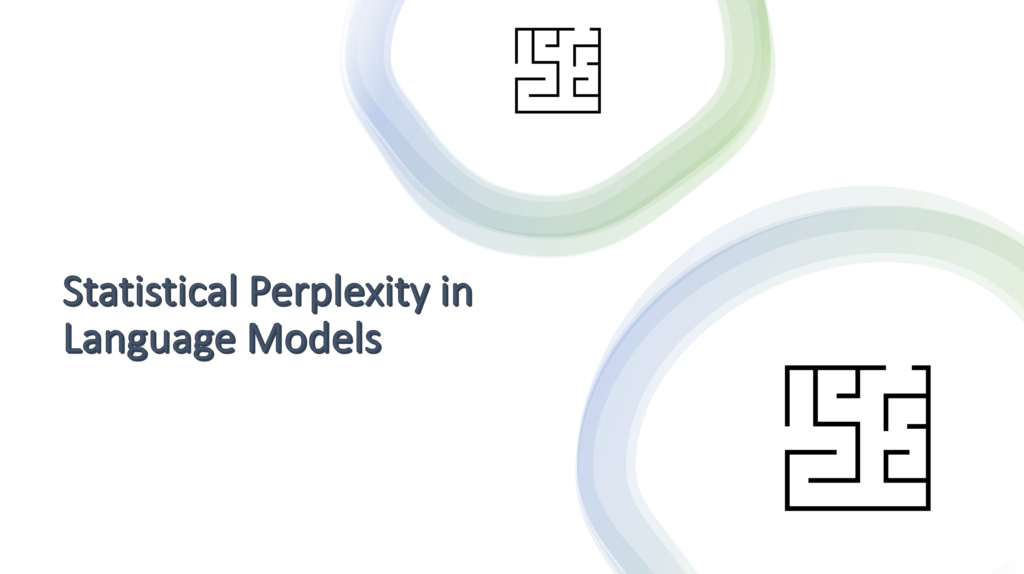## Perplexity: assessing the relevance of a text within a contextPerplexity is a statistical indicator that gauges the level of certainty a language model possesses in predicting text. This measurement essentially quantifies the model’s degree of «surprise» when it encounters previously unseen data. When the perplexity value decreases, it signifies an improved predictive prowess of the model, pointing to a more cohesive correspondence between the text and the training corpus.

In this example, we leverage the pretrained model «dmis-lab/biobert-base-cased-v1.2» (available in Hugging Face) to compute perplexity scores across a range of texts. The goal is to identify the relationship of each text with the biomedical context.

These are some possible scenarios where the perplexity measure can be used:

• Data cleansing to distinguish texts irrelevant to a specific field.
• Evaluate the fluency and coherence of the generated text.
• Identify outlier text within the dataset.
• Detects grammatical or terminological inaccuracies that require rectification.

In this Python script, the loss of the model’s predictions for the given text is calculated, followed by the computation of perplexity using the formula exp(loss / num_tokens).

Reference

https://en.wikipedia.org/wiki/Perplexity